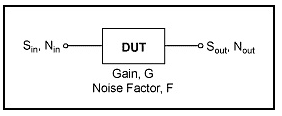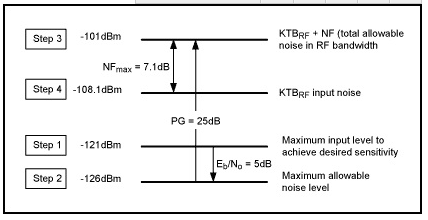# 加拿大28在线预测55新闻资讯

# 扩频系统的接收机灵敏度方程

## 从噪声系数F推导Eb/No关系1.

Sin = 可获得的输入信号功率(W)
Nin =
可获得的输入热噪声功率(W) = KTBRF其中:
K =
波尔兹曼常数 = 1.381 10-23 W/Hz/K,
T = 290K
，室温
BRF =
射频载波带宽(Hz) = 扩频系统的码片速率
Sout =
可获得的输出信号功率(W)
Nout =
可获得的输出噪声功率(W)
G =
设备增益(数值)
F =
设备噪声系数(数值)

F = (Sin / Nin) / (Sout / Nout) = (Sin / Nin) (Nout / Sout)

Nout = (F Nin Sout) / Sin其中Sout = G Sin

Nout = F Nin G

1 / T = 用户数据比特率, Rbit单位Hz, 得出Sin = Eb Rbit

Sout / Nout = (Sin G) / (Nin G F) =
Sin / (Nin F) =
(Eb Rbit) / (KTBRF F) =
(Eb/ KTF) (Rbit / BRF),

Sout / Nout = Eb/No Rbit / BRF

PG = BRF / Rbit

Sout / Nout = Eb/No 1 / PG.

## 接收机灵敏度方程

F = (Sin / Nin) / (Sout / Nout)F = (Sin / Nin) (Nout / Sout)
Sin = F Nin (Sout / Nout)

Sin又可以表示为：

Sin = F KTBRF Eb/No 1/PG

Sin (dBm) = NF (dB) + KTBRF (dBm) + Eb/No (dB) - PG (dB)

## 数字实例

• 对于速率为12.2kbps、功率-121dBm的数字语音信号，最大规定输入信号电平必须满足系统的最小规定灵敏度。
• 对于QPSK调制信号，在Eb/No值为5dB时可以获得规定的误码率BER (0.1%)
• 射频带宽等于码片速率，即3.84MHz
• KTBRF(log) = 10 log(1.381 10-23 W/Hz/K 290K 3.84MHz 1000mW/W) = -108.13dBm.
• 规定的用户数据速率Rbit等于12.2kbpsPGPG = Rchip / Rbit = 314.75numeric25dBlog
• 将这些值带入并利用等式：Sout / Nout = Eb/No Rbit / BRF得到输出信噪比为：5dB - 25dB = -20dB。这表示扩展了带宽的扩频系统实际是在负值SNR下工作。

Sin (dBm) = NF (dB) + KTBRF (dBm) + Eb/No (dB) - PG (dB)2.

## 小结

Sin (dBm) = NF (dB) + KTBRF (dBm) + Eb/No (dB) - PG (dB)

## 参考文献

1.     CDMA Systems Engineering Handbook, Jhong Sam Lee & Leonard E. Miller, Artech House Publishers, 1998.

2.     CDMA RF System Engineering, Samuel C. Yang, Artech House Publishers, 1998.

来源于网络资源，备忘。发表评论新闻资讯

021-51978216